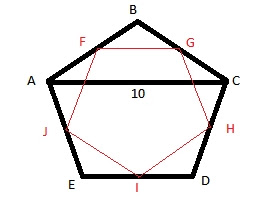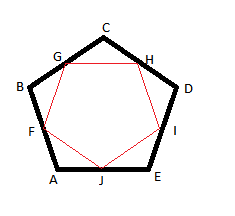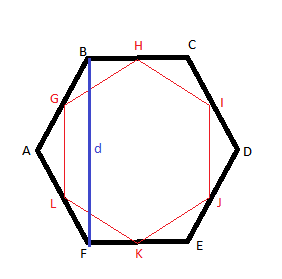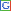## Thursday, January 12, 2012

### An Interesting Geometrical Puzzle

My daughter recently brought home a puzzle that had me scratching my head for a while before being able to solve it. Actually, the solution was quite simple once I figured it out, but it spawned off a different puzzle in my head that may be a little trickier. In any case, I have not figured out a solution to the spawned-off puzzle yet, but let me not get ahead of myself. Let me first tell you about the puzzle that my daughter brought home.You are given a regular pentagon, ABCDE (regular in this context means that all the sides are of equal length, and all the interior angles are equal to 108 degrees). You are also given the fact that the diagonal AC of this pentagon is 10 units long. Please refer to the figure on the left.

Now, inside this pentagon is another pentagon formed by joining the midpoints of each of the sides of the original pentagon. Call this inscribed pentagon FGHIJ. The puzzle is to use all this information to find the perimeter of the pentagon FGHIJ.

Initially, for some reason, I thought the solution would consist of finding the perimeter of ABCDE first and then somehow using that along with the length of AC to derive the perimeter of FGHIJ. That is what had me floundering for a few minutes. In fact, I even looked up the Wikipedia article on pentagons for some inspiration.

But the solution is actually much simpler (obviously, the fact that this puzzle was given to a middle-school student as an assignment is a big hint that this is not the geometric equivalent of Fermat's last theorem!). It is simply sufficient to realize that the diagonal creates a triangle (ABC in this case), and the line segment FG connects the midpoints of two of the sides of this triangle.

You can then apply the midpoint theorem on this triangle to conclude that side FG must be equal to 5 units in length because AC is 10 units long. And because FGHIJ is a regular pentagon, being the midpoint pentagon of a regular pentagon, its perimeter must be 5*5 = 25 units.

But, obviously, half the fun of solving a mathematical problem is coming up with related puzzles and questions. In particular, the puzzle I am now stuck with is as follows: what is the ratio of perimeters of midpoint polygons to the perimeters of the polygons they are inscribed in as a function of the number of sides of the polygon? Similarly, what is the ratio of areas of midpoint polygons to the perimeters of the polygons they are inscribed in as a function of the number of sides of the polygon?

Note that the polygons formed by joining the midpoints of the sides of a given polygon are called midpoint polygons, and have been studied by mathematicians in some detail. In particular midpoint polygons constructed from regular polygons are also regular, and are geometrically similar to the exterior polygon.Let us take a regular triangle (also known as an equilateral triangle) first. Connecting the midpoints of the three sides to create an inscribed midpoint triangle inside the original triangle gives us a triangle with half the perimeter as the original. Note that in the figure below, triangle ADF is also equilateral, and since AD and AF are one half of the length of AB, DF is one-half of the length of AB. And since DE, EF and DF have the same length (each equal to half the length of the sides of the original triangle ABC), the new triangle has half the perimeter as the original triangle.

Also note that the original triangle ABC has been divided into 4 equilateral triangles of equal area by the addition of the inscribed triangle DEF. Thus, the area of triangle DEF is one quarter of the area of the original triangle ABC. Thus, the ratio of perimeters is 1/2, and the ratio of areas is 1/4.Moving on to a square, which is a regular quadrilateral, we can use the pythagorean theorem on triangle EBF to figure out that the length of EF is equal to (length of AB)/sqrt(2). And the area of EFGH is one-half of the area of ABCD. Thus, the ratio of perimeters is 1/sqrt(2), and the ratio of areas is 1/2.The situation becomes more complicated in the case of a pentagon. In fact, it is not easy to figure out what the length of each side of an inscribed pentagon is if you know just the lengths of the sides of the original pentagon. But my research into regular pentagons on the Wikipedia site did have a positive side-effect: it turns out that the diagonals of a regular pentagon are in golden ratio to its side. In other words, if the length of a side is 1 unit, the length of a diagonal is eaual to the golden ratio. The value of golden ratio is 1.6180339887498948482045868343656.

From my solution of the puzzle which started this entire exploration, we also know that the length of each side of the inscribed pentagon is one half of the length of the diagonal. Thus, if the length of each original side is 1, then each diagonal is 1.6180339887498948482045868343656 units long, and the length of each inscribed side is 1.6180339887498948482045868343656/2 units long. Thus, the ratio of perimeters is 1.6180339887498948482045868343656/2.

The same Wikipedia article also tells me that the area of a regular pentagon with sides of length t is 1.720477401*t^2. Thus, the area of the inscribed pentagon would be 1.720477401*(1.6180339887498948482045868343656*t/2)^2. What we are interested in is the ratio of the areas, not the actual areas themselves. And that would simply be (1.6180339887498948482045868343656/2)^2.What about a hexagon? Well, the analysis is a little more complicated in the case of a hexagon. First of all there are two formulas for the area of a hexagon that we can use to derive the length of a diagonal of the hexagon that connects two alternate vertices of the hexagon (note that a hexagon also has three diagonals that connect opposite vertices, and these diagonals are twice the length of each side. They are also longer than the diagonals we are interested in). You can find these formulas in the wikipedia article on hexagons.

The first formula for area is: area = 2.598076211*t^2. The second formula for area is: area = 1.5*d*t. Interestingly, d is the length of the diagonal connecting alternate vertices of the hexagon (it is the height of the hexagon when it is resting on one of the sides as its base). Using these two formulas, since the area of a hexagon is a single number, we can say that 2.598076211*t^2 = 1.5*d*t. From this, we can derive the value of d to be 2.598076211*t/1.5.

At this point, we can then use the midpoint theorem to say that each side of an inscribed hexagon would be one-half the length of each of these diagonals. Thus the ratio of perimeters would be 2.598076211/3. And the ratio of areas would be the square of that number since the area is directly proportional to the square of the length of a side.

So, that is as far as I have gotten so far. The area formula for heptagons does not allow me to calculate the length of a diagonal connecting alternate vertices of a heptagon. So, I can not calculate the length of a side of an inscribed midpoint heptagon either. You could say, I am stuck!

In table form, one can express this as below:

 Number of Sides Ratio of perimeter of midpoint polygon to perimeter of exterior polygon Ratio of area of midpoint polygon to area of exterior polygon 3 0.50 0.25 4 0.70710678118654752440084436210485 0.50 5 0.8090169943749474241022934171828 0.65450849718747371205114670859138 6 0.86602540366666666666666666666667 0.75

Pretty basic observations follow from this.
• For regular polygons, area seems to be always proportional to the square of the length of a side. Thus, the ratio of areas of inscribed midpoint polygons to exterior polygons would be the square of the ratio of perimeters.
• The ratio of perimeters (and thus the areas) approaches 1, and will be 1 in the asymptotic case of a polygon with infinite number of sides (one could argue that the inscribed midpoint circle of a given circle is coincident with the given circle, and thus the ratio of perimeters as well as areas is indeed 1).
• Since the ratio of areas is the square of the ratio of perimeters, and the ratio of perimeters is less than 1.0, the ratio of areas is always less than the ratio of perimeters.

These observations though, do not tell me the answer to the question I started with: what is the ratio of the perimeter of an inscribed midpoint polygon to the perimeter of the exterior polygon? Is it possible to find an expression that tells me the value of this ratio for any type of polygon (obviously, such an expression would be a function of the number of sides of the polygon)? Any ideas? I would love to hear your thoughts on how to proceed with this investigation. Thank you, and good luck!Anonymous said...

whoah this weblog is fantastic i really like studying your posts. Keep up the great work! You already know, lots of persons are looking round for this info, you can aid them greatly.
Tiffen Lens Cleaning Tissue, 50 Packs of 50 Sheets EachAnonymous said...

electronic cigarette starter kit, smokeless cigarettes, smokeless cigarettes, electronic cigarette, electronic cigarettes, electronic cigarettes

## Content From TheFreeDictionary.com

In the News

Article of the Day

This Day in History

Today's Birthday

Quote of the Day

Word of the Day

Match Up
 Match each word in the left column with its synonym on the right. When finished, click Answer to see the results. Good luck!

Hangman

Spelling Beedifficulty level:
score: -